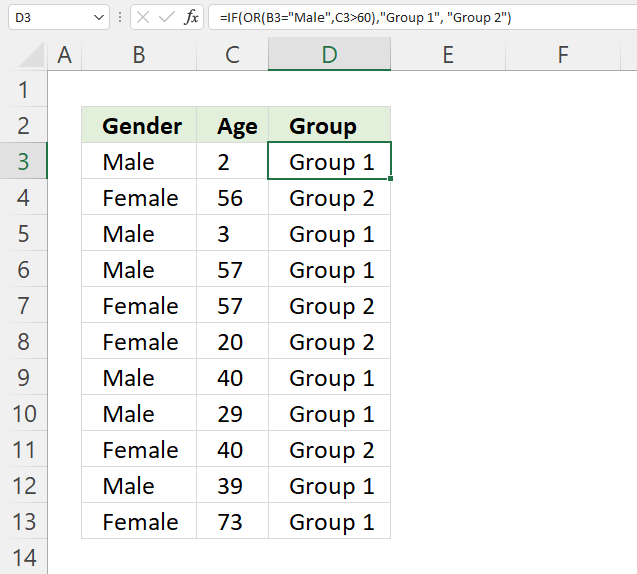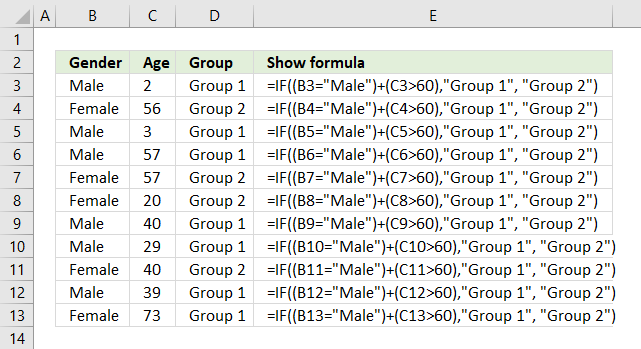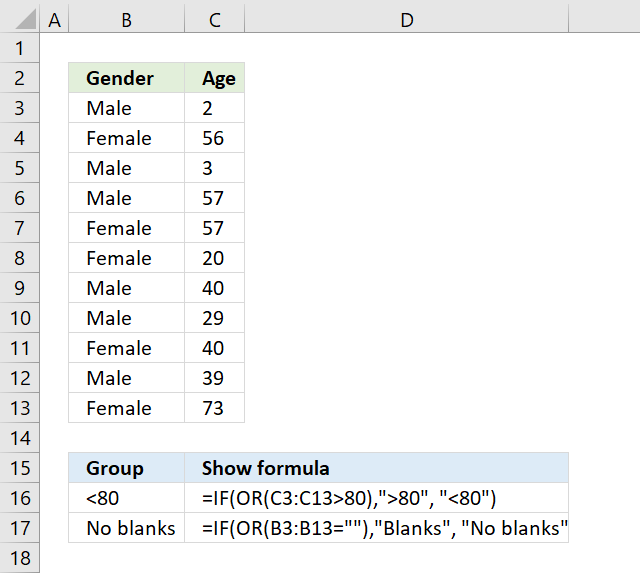Author: Oscar Cronquist Article last updated on October 26, 2021This article shows examples of how to use the IF function with the OR function.

## 1. IF with OR functionThe formula above in cell D3 evaluates two different logical tests, if at least one of them is TRUE one thing happens, if all return FALSE another thing happens.

=IF(OR(B3="Male",C3>60),"Group 1", "Group 2")

If a value in column B is equal to "Male" or a value on the same row in column C is above 60 the formula returns "Group 1", if none of these logical expressions return TRUE the formula returns "Group 2"

### 1.1 Explaining formula in cell D3

#### Step 1 - First condition

The equal sign is a logical operator, it lets you check if a value is equal to a condition. It returns a boolean value TRUE or FALSE.

B3="Male"

becomes

"Male"="Male"

and returns TRUE. Note that the comparison is not case sensitive, use the EXACT function if you want that functionality.

#### Step 2 - Second condition

The larger than sign is a also logical operator, it lets you check if a value is larger than a condition. It returns a boolean value TRUE or FALSE.

C3>60

becomes

2>60

and returns FALSE. 2 is not larger than 60.

#### Step 3 - Evaluate OR function

The OR function returns TRUE or FALSE based on a logical expression. It returns TRUE if at least one argument evaluates to TRUE, the OR function returns FALSE if all arguments return FALSE.

OR(B3="Male",C3>60)

becomes

OR(TRUE, FALSE)

and returns TRUE.

#### Step 4 - Evaluate IF function

The IF function returns one value if the logical test is TRUE and another value if the logical test is FALSE.

IF(logical_test, [value_if_true], [value_if_false])

IF(OR(B3="Male",C3>60),"Group 1", "Group 2")

becomes

IF(TRUE, "Group 1", "Group 2")

and returns "Group 1" in cell D3.

### 1.2 OR function or plus sign?You can also wrap each logical expression with parentheses and use the + (plus sign) with the same functionality as the OR function.

=IF((B3="Male")+(C3>60),"Group 1", "Group 2")

Why wrap each logical expression? It determines the order of calculation, you want the comparisons to be calculated before you add the boolean values.

You now know what a plus sign means if you happen to see a formula with a plus sign. The advantage is a slightly smaller formula.

### Explaining formula

#### Step 1 - First condition

The equal sign is a logical operator, it lets you check if a value is equal to a condition. It returns a boolean value TRUE or FALSE.

B3="Male"

becomes

"Male"="Male"

and returns TRUE.

#### Step 2 - Second condition

The larger than sign is a also logical operator, it lets you check if a value is larger than a condition. It returns a boolean value TRUE or FALSE.

C3>60

becomes

2>60

and returns FALSE. 2 is not larger than 60.

#### Step 3 - Add boolean values - OR logic

(B3="Male")+(C3>60)

becomes

TRUE + FALSE

and returns 1. 1 is the numerical equivalent to TRUE and 0 (zero) is FALSE.

#### Step 4 - Evaluate IF function

The IF function returns one value if the logical test is TRUE and another value if the logical test is FALSE.

IF(logical_test, [value_if_true], [value_if_false])

IF((B3="Male")+(C3>60),"Group 1", "Group 2")

becomes

IF(TRUE, "Group 1", "Group 2")

and returns "Group 1" in cell D3.

## 2. IF with OR function - check if there is at least one number above a given valueThe array formula in cell B16 checks if there is a value larger than 80 in cell range C3:C13. You need to enter this as an array formula because you carry out a logical test to each cell in cell range C3:C13 and that logical test returns an array of boolean values.

=IF(OR(C3:C13>80),">80", "<80")

The OR function then returns TRUE if at least one boolean value is TRUE in the array or else FALSE. The IF function returns <80 if there are no values above 80 and >80 if there is at least one value above 80 in cell range C3:C13.

### Explaining formula in cell B16

#### Step 1 - Check if values in cell range are larger than 80

The larger than sign is a also logical operator, it lets you check if a value is larger than a condition. It returns a boolean value TRUE or FALSE.

C3:C13>80

#### Step 2 - Apply OR logic

The OR function returns TRUE or FALSE based on a logical expression. It returns TRUE if at least one argument evaluates to TRUE, the OR function returns FALSE if all arguments return FALSE.

OR(C3:C13>80)

#### Step 3 - Evaluate IF function

The IF function returns one value if the logical test is TRUE and another value if the logical test is FALSE.

IF(logical_test, [value_if_true], [value_if_false])

C3:C13>80

## 3. How to check if there is a blank value in a cell rangeThe array formula in cell B17 checks if there are any blank values in cell range B3:B13.

=IF(OR(B3:B13=""),"Blanks", "No blanks")

### 3.1 How to enter an array formula

To enter an array formula press and hold CTRL + SHIFT simultaneously, then press Enter once. Release all keys.

The formula bar now shows the formula enclosed with curly brackets telling you that you entered the formula successfully. Don't enter the curly brackets yourself.

### 3.2 Explaining formula

#### Step 1 - Check if cells are empty

The equal sign is a logical operator, it lets you check if a value is equal to a condition. It returns a boolean value TRUE or FALSE.

B3:B13=""

#### Step 2 - Apply Or logic

The OR function returns TRUE or FALSE based on a logical expression. It returns TRUE if at least one argument evaluates to TRUE, the OR function returns FALSE if all arguments return FALSE.

OR(B3:B13="")

#### Step 3 - Evaluate IF function

The IF function returns one value if the logical test is TRUE and another value if the logical test is FALSE.

IF(logical_test, [value_if_true], [value_if_false])

IF(OR(B3:B13=""),"Blanks", "No blanks")

## 4. Get Excel *.xlsx file

IF with OR function.xlsx